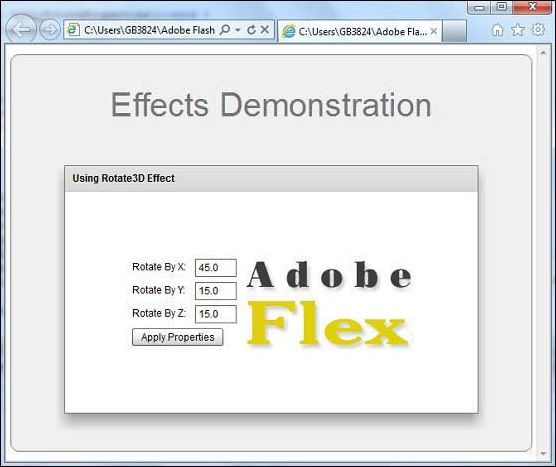# Flex - Rotate3D Effect

## Introduction

The Rotate3D class rotate a target object in three dimensions around the x, y, or z axes. The rotation occurs around the transform center of the target.

## Class Declaration

Following is the declaration for spark.effects.Rotate3D class −

```public class Rotate3D
extends AnimateTransform3D
```

## Public Properties

Sr.No Property & Description
1

angleXFrom : Number

The starting angle of rotation of the target object around the x axis, expressed in degrees.

2

angleXTo : Number

The ending angle of rotation of the target object around the x axis, expressed in degrees.

3

angleYFrom : Number

The starting angle of rotation of the target object around the y axis, expressed in degrees.

4

angleYTo : Number

The ending angle of rotation of the target object around the y axis, expressed in degrees.

5

angleZFrom : Number

The starting angle of rotation of the target object around the z axis, expressed in degrees.

6

angleZTo : Number

The ending angle of rotation of the target object around the z axis, expressed in degrees.

## Public Methods

Sr.No Method & Description
1

Rotate3D(target:Object = null)

Constructor.

## Methods Inherited

This class inherits methods from the following classes −

• spark.effects.AnimateTransform3D
• spark.effects.AnimateTransform
• spark.effects.Animate
• mx.effects.Effect
• flash.events.EventDispatcher
• Object

## Flex Rotate3D Effect Example

Let us follow the following steps to check usage of Rotate3D Effect in a Flex application by creating a test application −

Step Description
1 Create a project with a name HelloWorld under a package com.tutorialspoint.client as explained in the Flex - Create Application chapter.
2 Modify HelloWorld.mxml as explained below. Keep rest of the files unchanged.
3 Compile and run the application to make sure business logic is working as per the requirements.

Following is the content of the modified mxml file src/com.tutorialspoint/HelloWorld.mxml.

```<?xml version = "1.0" encoding = "utf-8"?>
width = "100%" height = "100%" minWidth = "500" minHeight = "500">

<fx:Style source = "/com/tutorialspoint/client/Style.css" />
<fx:Script>
<![CDATA[
private function applyRotateProperties():void {
rotateEffect.play();
}
]]>
</fx:Script>

<fx:Declarations>
<s:Rotate3D id = "rotateEffect" target = "{imageFlex}"
angleXFrom = "0.0" angleXTo = "{Number(rotateX.text)}"
angleYFrom = "0.0" angleYTo = "{Number(rotateY.text)}"
angleZFrom = "0.0" angleZTo = "{Number(rotateZ.text)}"
/>
</fx:Declarations>

<s:BorderContainer width = "630" height = "480" id = "mainContainer"
styleName = "container">
<s:VGroup width = "100%" height = "100%" gap = "50"
horizontalAlign = "center" verticalAlign = "middle">
<s:Label id = "lblHeader" text = "Effects Demonstration"
fontSize = "40" color = "0x777777" styleName = "heading" />

<s:Panel id = "rotate3DPanel" title = "Using Rotate3D Effect"
width = "500" height = "300">
<s:layout>
<s:HorizontalLayout  gap = "10" verticalAlign = "middle"
horizontalAlign = "center" />
</s:layout>

<s:VGroup top = "10" left = "15">
<s:HGroup verticalAlign = "middle">
<s:Label text = "Rotate By X:" width = "70" />
<s:TextInput id = "rotateX" text = "45.0" width = "50" />
</s:HGroup>

<s:HGroup verticalAlign = "middle">
<s:Label text = "Rotate By Y:" width = "70" />
<s:TextInput id = "rotateY" text = "15.0" width = "50" />
</s:HGroup>

<s:HGroup verticalAlign = "middle">
<s:Label text = "Rotate By Z:" width = "70" />
<s:TextInput id = "rotateZ" text = "15.0" width = "50" />
</s:HGroup>

<s:Button label = "Apply Properties"
click = "applyRotateProperties()" />
</s:VGroup>

<s:Image id = "imageFlex"
source = "http://www.tutorialspoint.com/images/flex-mini.png"
height = "200" width = "200" />
</s:Panel>
</s:VGroup>
</s:BorderContainer>
</s:Application>
```

Once you are ready with all the changes done, let us compile and run the application in normal mode as we did in Flex - Create Application chapter. If everything is fine with your application, it will produce the following result: [ Try it online ]flex_visual_effects.htm